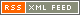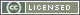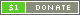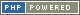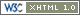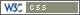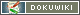# Number

A Number is any whole or decimal, positive or negative number. It is further categorised into Integers ("whole" numbers), and Floats (a floating-point, or real, number). Numbers can only be specified in base-10 (decimal). They can be preceded by a sign symbol (`+` or `-`) to set the sign of the number (note that the sign symbols are also the Number typecast operators).

For example:

```{ a = 123 // An integer }
{ a =   0 // An integer }
{ a =  -4 // An integer }

{ b = 1.45 // A float }
{ b = 0.0  // A float }
{ b = -9.6 // A float }```

Numbers in standard form, such as `-5.4e7` and `4E-11` can be used by converting them from strings, for instance `+"4E-11"`. See Converting to Number below.

### Converting to Number

To explicitly convert a value to Number, the Number typecast operator - `+` - should be used (`+` converts as-is, whereas `-` converts to Number and also reverses the sign). Some operators, such as the numerical multiplication operator - `*` - also explictly convert a value to Number.

When converting to Number:

• Boolean `false` is converted to `0`, and `true` is converted to `1`
• Strings will be converted to Floats if the string contains a number in standard form, or a number with a decimal point in it. In all cases, the first part of the string is used for the conversion, which stops when non-numerical data is found.
• Arrays are currently converted to the numerical equivalent of their Boolean value. In the future this may well change to give the number of items in the Array.